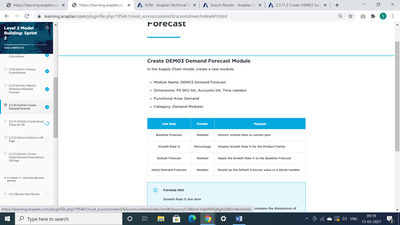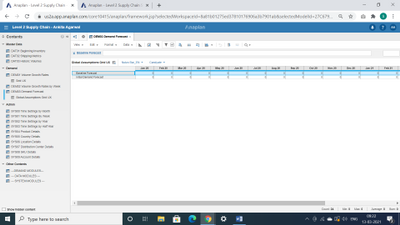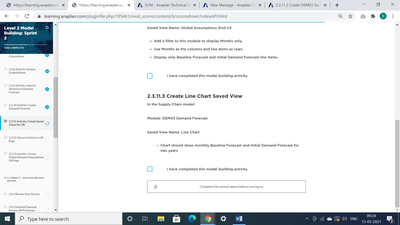# 2.3.11.3 Create Line Chart Saved ViewHi , I am very confused on Level 2 Sprint 2 module.Here in the above screenshot we are just creating the formula for Growth Rate%  , rest everything remains zero.

But then next steps we are asked to create saved views based on Baseline forecast and Initial demand forecast. (Global Assumptions Grid UX)Am I missing something ?

The Line chart view is also based on thisRegards,

Ankita

•I didn’t understand the formula so well. I will try again .

also what about default forecast and initial demand forecast formulas.
I got the formula for growth rate .

•Thanks a lot for helping me out . But one big question which activity it is telling us to create these formulas. Only growth rate formula was told .

•No worries. You'll be building that soon - you've got a head start. You got this!

•Thank you
•Hi

I am struggling to find out the formulas for

• Final Forecast
• Forecast CF